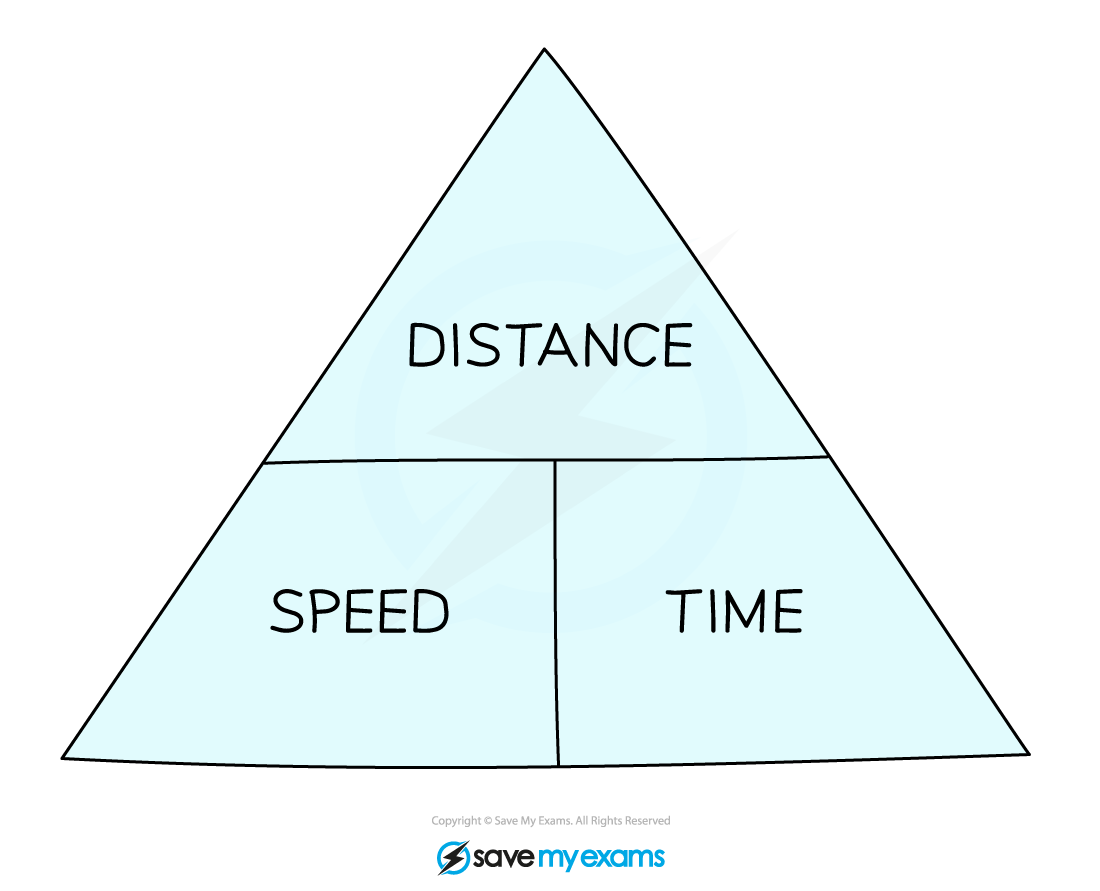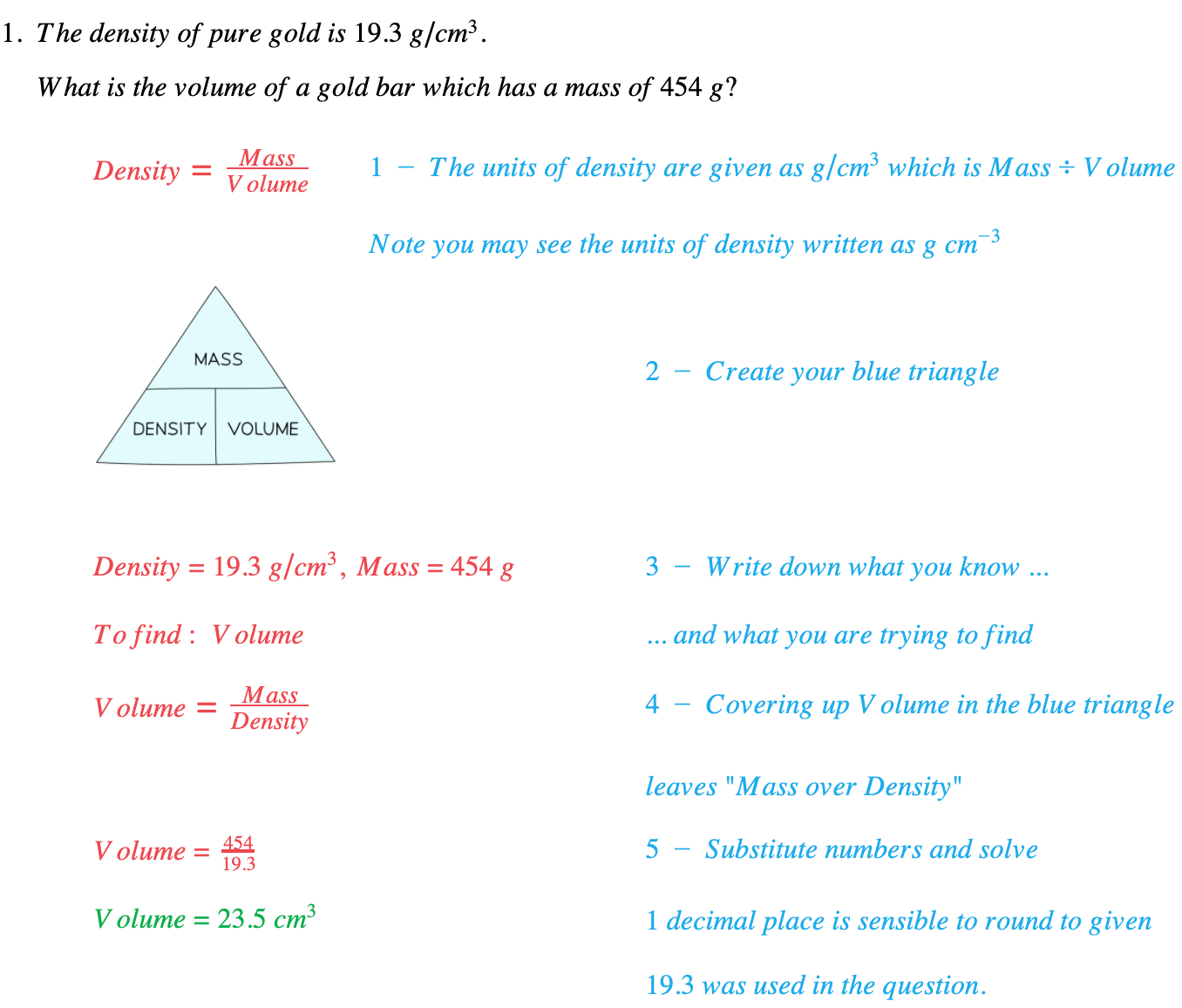# Edexcel IGCSE Maths 复习笔记 4.4.1 Speed, Density & Pressure

Edexcel IGCSE Maths 复习笔记 4.4.1 Speed, Density & Pressure

#### What connects speed, density & pressure?

• Speed, density and pressure are all examples of variables which are calculated by dividing one thing by another:Speed = Distance ÷ Time

Density = Mass ÷ VolumePressure = Force ÷ Area

• In that respect they can all be treated in the same way

#### Doing speed, density & pressure questions

1. Use UNITS in Q (or other info) to write down a formula

2. Create BLUE TRIANGLE:e.g. for Speed, Distance and Time3. For each part of the Q write down

what you knowwhat you want to know

4. Use Blue Triangle to REARRANGE formula (if necessary)5. SUBSTITUTE numbers and SOLVE

#### Worked Example